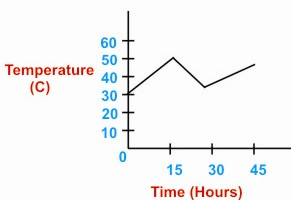HOME MATH DICTIONARY DOWNLOAD FEEDBACK DISCLAIMER
 Question: What do you mean by Line Graph ? Answer: Line graphs compare two variables. Each variable is plotted along an axis . A line graph has a vertical axis and a horizontal axis. For example, if you wanted to graph the height of a ball after you have thrown it, you could put time along the horizontal, or x-axis, and height along the vertical, or y-axis. Example of a Line Graph: